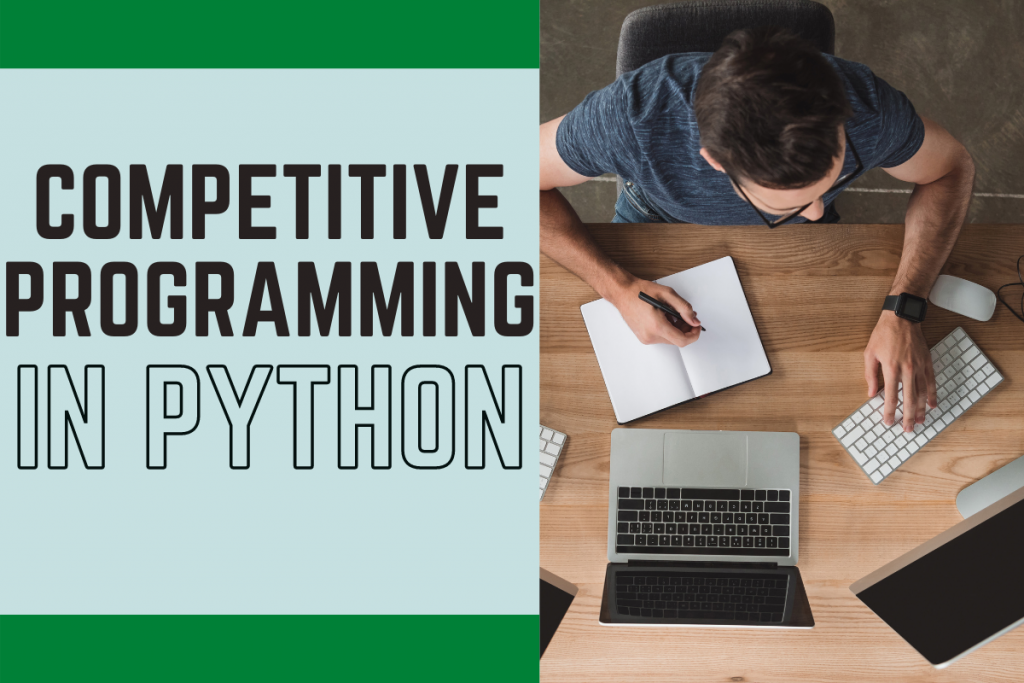# Competitive Programming in Python: What you need to know?Hello, there coder! I am pretty sure you are familiar with what Competitive Programming is. But there are some important things one needs to keep in mind when coding in python. These small things can create a huge difference in your code.

## Competitive Programming in Python

Let’s study a few of them one after another.

### 1. Use of Generators

The use of Generators can end up reducing both space and time complexities and are better than using functions. An illustration of a generator function is shown below.

It is also helpful in returning multiple values one after another at the same time.

```def FirstGen():
yield 1
yield 2
yield 3
for i in FirstGen():
print(i,end=" ")
```

### 2. Use of Built-in Functions

Using built-in functions and libraries is a better approach than the normal approach. Let us look at a simple program below to have a new list that contains the square of elements of the first list.

To illustrate the difference better, we will calculate the execution time of the programs as well with the help of the time `time` module.

```import time
start_time = time.time()

def get_square(x):
return x**2
l1 = [i for i in range(100000)]
l2 = []
for i in l1:
l2.append(get_square(i))
print(len(l2))

print("Time taken by the code: %s seconds."% (time.time() - start_time))
```

The above approach shows the correct output in `0.06881594657897949` seconds which is no doubt decent enough.

Now let’s try the same program using the in-built function, `map` and apply the function declared to the list directly.

```import time
start_time = time.time()

def get_square(x):
return x**2
l1 = [i for i in range(100000)]
l2 = list(map(get_square,l1))
print(len(l2))

print("Time taken by the code: %s seconds."% (time.time() - start_time))
```

Here we saw that the time taken by the same list is `0.048911094665527344` seconds which might seem like a very minor difference but for even larger data this difference can tend to get bigger.

### 3. Using itertools

The itertools the module can be really helpful to solve some complex problems. For instance, look at the program given below to find all permutations of a list.

```import itertools
x = list(itertools.permutations([1,2,3]))
print(x)
```

The same can be done by creating your own logic and functions but that would be way too complex and will have a worse time complexity.

### 4. Using map function

Whenever we are required to take input of all the elements of an integer array in one single line separated by white spaces, the map function is the best shot in achieving the same.

```l1 = list(map(int,input("Enter all the elements: ").split()))
print(l1)
```

Using the `map` function simplifies the complexity of handling multiple values being entered in a single line.

### 5. String Concatenation

To concatenate multiple strings together we can use two approaches: Adding strings to strings or using join function.

Using `join` function is recommended as it performs the whole concatenation process in a single line and decreases the complexity if the no of strings is huge.

Let’s look at the first approach: Using the addition operation on strings. The program given below ends up having `0.00498509407043457` seconds as the execution time.

```import time
start_time = time.time()
l = [str(i) for i in range(10000)]
st=""
for i in l:
st+=i
print(len(st))
print("Time taken by the code: %s seconds."% (time.time() - start_time))
```

Whereas, the second approach: Using the join operation gives a time complexity of just `0.002988576889038086` seconds which is obviously lesser.

```import time
start_time = time.time()
l = [str(i) for i in range(10000)]
st = ""
st.join(l)
print(len(st))
print("Time taken by the code: %s seconds."% (time.time() - start_time))
```

## Conclusion

Congratulations! Today you learned some very basic but important things to keep in mind when doing competitive programming in the python programming language.

The tricks can surely help you in increasing the efficiency and accuracy of our solutions to a great extent.

Try out by yourself! Happy coding!